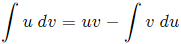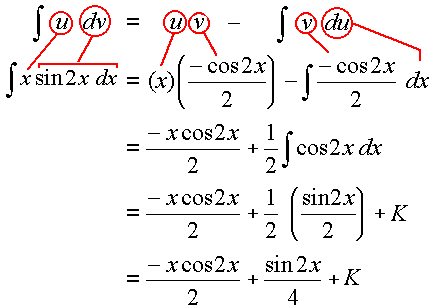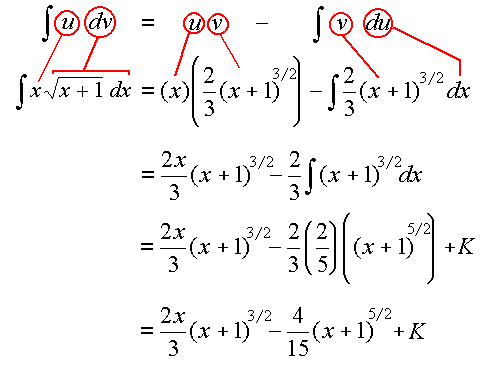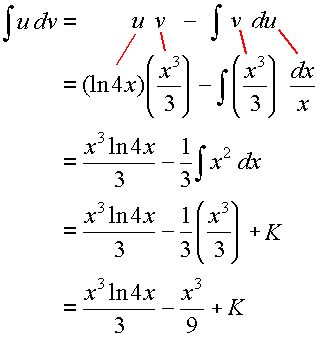Search IntMath
Close

450+ Math Lessons written by Math Professors and Teachers

5 Million+ Students Helped Each Year

1200+ Articles Written by Math Educators and Enthusiasts

Simplifying and Teaching Math for Over 23 Years

# 7. Integration by Parts

by M. Bourne

Sometimes we meet an integration that is the product of 2 functions. We may be able to integrate such products by using Integration by Parts.

If u and v are functions of x, the product rule for differentiation that we met earlier gives us:

d/(dx)(uv)=u(dv)/(dx)+v(du)/(dx)

Rearranging, we have:

u(dv)/(dx)=d/(dx)(uv)-v(du)/(dx)

Integrating throughout with respect to x, we obtain the formula for integration by parts:This formula allows us to turn a complicated integral into more simple ones. We must make sure we choose u and dv carefully.

NOTE: The function u is chosen so that (du)/(dx) is simpler than u.

## Priorities for Choosingu

When you have a mix of functions in the expression to be integrated, use the following for your choice of u, in order.

1. Let u = ln x

2. Let u = x^n

3. Let u = e^(nx)

### Example 1

intx\ sin 2x\ dx

#### Solution

We need to choose u. In this question we don't have any of the functions suggested in the "priorities" list above.

We could let u = x or u = sin 2x, but usually only one of them will work. In general, we choose the one that allows (du)/(dx) to be of a simpler form than u.

So for this example, we choose u = x and so dv will be the "rest" of the integral, dv = sin 2x dx.

We have u = x so du = dx.

Also dv = sin 2x\ dx and integrating gives:

v=intsin 2x\ dx

=(-cos 2x)/2

Substituting these 4 expressions into the integration by parts formula, we get (using color-coding so it's easier to see where things come from):

int \color{green}{\underbrace{u}}\ \ \ \color{red}{\underbrace{dv}}\ \   =\ \ \color{green}{\underbrace{u}}\ \ \ \color{blue}{\underbrace{v}} \ \ -\ \ int \color{blue}{\underbrace{v}}\ \ \color{magenta}{\underbrace{du}}

int \color{green}{\fbox{:x:}}\ \color{red}{\fbox{:sin 2x dx:}} = \color{green}{\fbox{:x:}}\ \color{blue}{\fbox{:{-cos2x}/2:}} - int \color{blue}{\fbox{:{-cos2x}/2:}\ \color{magenta}{\fbox{:dx:}}

 = (-xcos2x)/2 + 1/2 int cos2x dx

 = (-xcos2x)/2 + 1/2 (sin2x)/2 +K

 = (-xcos2x)/2 + (sin2x)/4 +K

If the above is a little hard to follow (because of the line breaks), here it is again in a different format:### Example 2

int x sqrt(x+1) dx

intxsqrt(x+1)\ dx

We could let u=x or u=sqrt(x+1).

Once again, we choose the one that allows (du)/(dx) to be of a simpler form than u, so we choose u=x.

Therefore du = dx. With this choice, dv must be the "rest" of the integral: dv=sqrt(x+1)\ dx.

u = x so du=dx.

dv=sqrt(x+1)\ dx, and integrating gives:

v=intsqrt(x+1) dx

=int(x+1)^(1//2)dx

=2/3(x+1)^(3//2)

Substituting into the integration by parts formula, we get:

int \color{green}{\underbrace{u}}\ \ \ \color{red}{\underbrace{dv}}\ \   =\ \ \color{green}{\underbrace{u}}\ \ \ \color{blue}{\underbrace{v}} \ \ -\ \ int \color{blue}{\underbrace{v}}\ \ \color{magenta}{\underbrace{du}}

int \color{green}{\fbox{:x:}}\ \color{red}{\fbox{:sqrt(x+1) dx:}} = \color{green}{\fbox{:x:}}\ \color{blue}{\fbox{:2/3(x+1)^(3//2):}}  - int \color{blue}{\fbox{:2/3(x+1)^(3//2):}\ \color{magenta}{\fbox{:dx:}}

 = (2x)/3(x+1)^(3//2) - 2/3 int (x+1)^{3//2}dx

 = (2x)/3(x+1)^(3//2)  - 2/3(2/5) (x+1)^{5//2} +K

 = (2x)/3(x+1)^(3//2)- 4/15(x+1)^{5//2} +K

Once again, here it is again in a different format:### Example 3

intx^2 ln 4x\ dx

intx^2ln\ 4x\ dx

We could let u=x^2 or u=ln\ 4x..

Considering the priorities given above, we choose u = ln\ 4x and so dv will be the rest of the expression to be integrated dv = x^2\ dx.

With u=ln\ 4x, we have du=dx/x.

Integrating dv = x^2\ dx gives:

v=intx^2dx=x^3/3

Substituting in the Integration by Parts formula, we get:

int \color{green}{\underbrace{u}}\ \ \ \color{red}{\underbrace{dv}}\ \   =\ \ \color{green}{\underbrace{u}}\ \ \ \color{blue}{\underbrace{v}} \ \ -\ \ int \color{blue}{\underbrace{v}}\ \ \color{magenta}{\underbrace{du}}

int \color{green}{\fbox{:x^2:}}\ \color{red}{\fbox{:ln 4x dx:}} = \color{green}{\fbox{:ln 4x:}}\ \color{blue}{\fbox{:x^3/3:}}  - int \color{blue}{\fbox{:x^3/3:}\ \color{magenta}{\fbox{:dx/x:}}

 = (x^3 ln 4x)/3 - 1/3 int x^2 dx

 = (x^3 ln 4x)/3  - 1/3 x^3/3 +K

 = (x^3 ln 4x)/3 - x^3/9 +K

Once again, here it is again in a different format:### Example 4

intx\ sec^2 x\ dx

int x\ sec^2 x\ dx

We choose u=x (since it will give us a simpler du) and this gives us du=dx.

Then dv will be dv=sec^2x\ dx and integrating this gives v=tan x.

Substituting these into the Integration by Parts formula gives:

int x\ sec^2 x\ dx =intu\ dv

=uv-intv\ du

=(x)(tan x)-int(tan x)dx

=x\ tan x-(-ln\ |cos x|)+K

=x\ tan x+ln\ |cos x|+K

### Example 5

intx^2e^(-x)dx

intx^2 e^-x dx

The 2nd and 3rd "priorities" for choosing u given earlier said:

2. Let u = x^n

3. Let u = e^(nx)

This questions has both a power of x and an exponential expression. But we choose u=x^2 as it has a higher priority than the exponential. (You could try it the other way round, with u=e^-x to see for yourself why it doesn't work.)

So u=x^2 and this gives du=2x\ dx.

That leaves dv=e^-x\ dx and integrating this gives us v=-e^-x.

We substitute these into the Integration by Parts formula to give:

intx^2 e^-x dx =intu\ dv

=uv-intv\ du

=x^2(-e^-x)-int(-e^-x)(2x\ dx)

=-x^2e^-x+2intxe^-x dx

Now, the integral we are left with cannot be found immediately. We need to perform integration by parts again, for this new integral.

This time we choose u=x giving du=dx.

Once again we will have dv=e^-x\ dx and integrating this gives us v=-e^-x.

Substituting into the integration by parts formula gives:

int x e^-x dx =intu\ dv

=uv-intv\ du

=x(-e^-x)-int(-e^-x)dx

=-xe^-x+inte^-x dx

=-xe^-x-e^-x

So putting this answer together with the answer for the first part, we have the final solution:

intx^2e^-xdx =-x^2e^-x+2(-xe^-x-e^-x)

=-e^-x(x^2+2x+2)+K

### Example 6

int ln x dx

int ln\ x\ dx

Our priorities list above tells us to choose the logarithm expression for u. (of course, there's no other choice here. :-)

So with u=ln\ x, we have du=dx/x.

Then dv will simply be dv=dx and integrating this gives v=x.

Subsituting these into the Integration by Parts formula gives:

int ln\ x\ dx=int u\ dv

=uv-intv\ du

=x\ ln\ x-intx(dx)/x

=x\ ln\ x-intdx

=x\ ln\ x-x+K

### Example 7

intarcsin x dx

Using integration by parts, we set:

u=arcsin x, giving du=1/sqrt(1-x^2)dx.

Then dv=dx and integrating gives us v=x.

We now use:

intu\ dv=uv-intv\ du

This gives us:

int arcsin x\ dx =x\ arcsin x-intx/(sqrt(1-x^2))dx

Now, for that remaining integral, we just use a substitution (I'll use p for the substitution since we are using u in this question already):

p = 1 − x^2

So dp=-2x\ dx

This will yield:

intx/(sqrt(1-x^2))dx =-1/2int(dp)/sqrtp

=-1/2(2sqrtp)+K

=-sqrt(1-x^2)+K

int arcsin x\ dx =x\ arcsin x-(-sqrt(1-x^2))+K

= \x\ arcsin x+sqrt(1-x^2)+K

This time we integrated an inverse trigonometric function (as opposed to the earlier type where we obtained inverse trigonometric functions in our answer). See Integration: Inverse Trigonometric Forms.

### Alternate Method for Integration by Parts

Here's an alternative method for problems that can be done using Integration by Parts. You may find it easier to follow.

## Problem SolverThis tool combines the power of mathematical computation engine that excels at solving mathematical formulas with the power of GPT large language models to parse and generate natural language. This creates math problem solver thats more accurate than ChatGPT, more flexible than a calculator, and faster answers than a human tutor. Learn More.